Question

# One professor grades homework by randomly choosing 3 out of 7 homework problems to grafe. If...

One professor grades homework by randomly choosing 3 out of 7 homework problems to grafe. If a student completed the selected problems, he or she will get a perfect score. Elise did 5 problems. What is the probability that she will get a perfect score.

Total selected problems by professor = 3

total assignments to be done by students = 7

total assignements Elise did =5

P( assignment completed by Elise will be chosen by professor in first attempt ) = 5/7

P(assignment completed by Elise will be chosen by professor in second attempt given completed assignment was chosen in first draw ) = 4/6

P(assignment completed by Elise will be chosen by professor in sthird attempt given completed assignment was chosen in first draw ) = 3/5

P( Elise get complete score) = probability that professor chose 3 assignments from those 5 assignements completed by Elise =   (5/7) * (4/6) * (3/5)

= 0.28571

So , there is 28.571 probability that Elise will get full grades

#### Earn Coins

Coins can be redeemed for fabulous gifts.

Similar Homework Help Questions
• ### one professor grades homework by randomly choosing 5 out of 12homework problems to grade. a.) how many different gro...

one professor grades homework by randomly choosing 5 out of 12homework problems to grade. a.) how many different groups of 5 problems can be chosen fromthe 12 problems? b.) probability extension: jerry did only 5 problems of oneassignment. what is the probability that the problems he didcomprised the group that was selected to be graded? c.) silvia did 7 problems. how many different groups of 5 didshe complete? what is the probability that one of the groups of 5she completed...

• ### A mathematics professor assigns two problems for homework and knows that the probability of a student...

A mathematics professor assigns two problems for homework and knows that the probability of a student solving the first problem is 0.75, the probability of solving the second is 0.50, and the probability of solving both is 0.25. (Round your answers to three decimal places.) (a) Jed has solved the second problem. What is the probability he also solves the first problem? (b) Edna has solved the first problem. What is the probability she also solves the second problem?

• ### 1. A statistics professor posted 10 review problems on probability distributions: 4 problems were on discrete...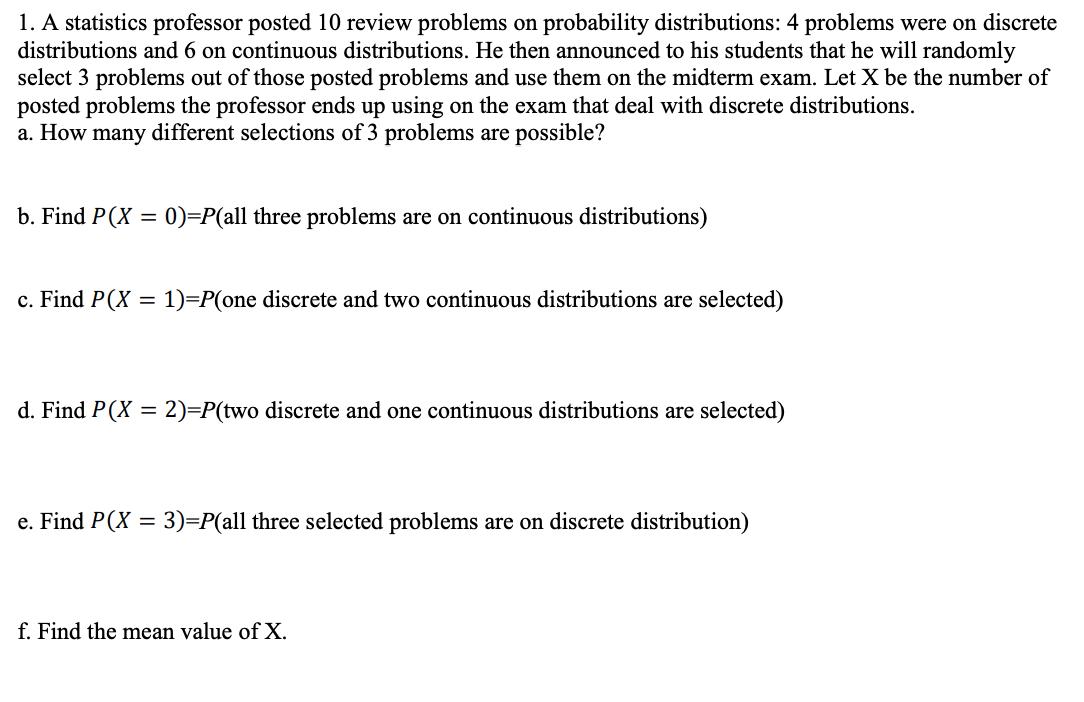1. A statistics professor posted 10 review problems on probability distributions: 4 problems were on discrete distributions and 6 on continuous distributions. He then announced to his students that he will randomly select 3 problems out of those posted problems and use them on the midterm exam. Let X be the number of posted problems the professor ends up using on the exam that deal with discrete distributions. a. How many different selections of 3 problems are possible? b. Find...

• ### Giving a test to a group of students, the grades and gender are summarized below Grades...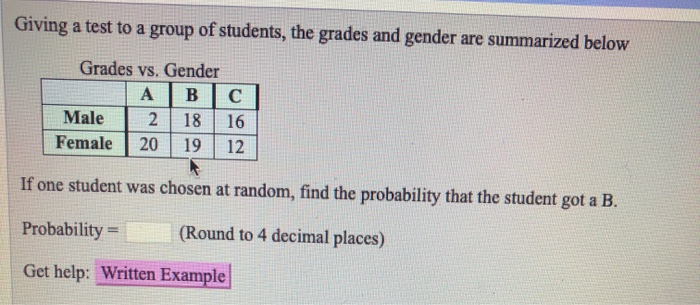Giving a test to a group of students, the grades and gender are summarized below Grades vs. Gender A | B | C Male 2 | 18 | 16 Female 2019 12 If one student was chosen at random, find the probability that the student got a B. Probability = (Round to 4 decimal places) Get help: Written Example Giving a test to a group of students, the grades and gender are summarized below Grades vs. Gender ABC Male 13...

• ### Homework: Chapter 3 Review Homework Save Score: 0.33 of 1 pt 13 of 38 (18 complete) HW Score: 31....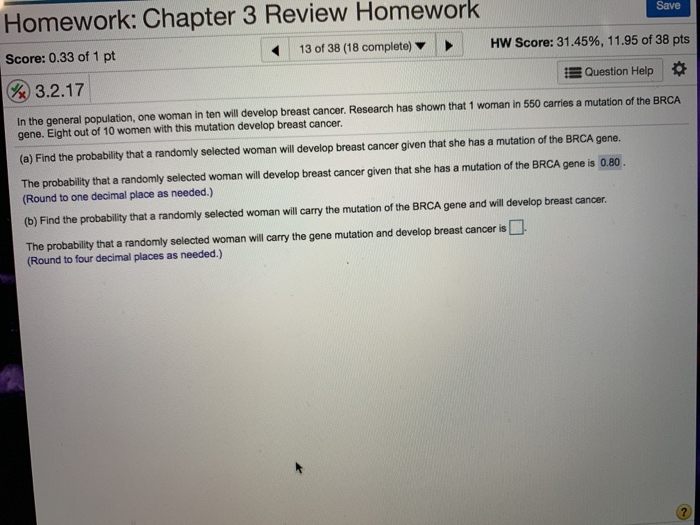Homework: Chapter 3 Review Homework Save Score: 0.33 of 1 pt 13 of 38 (18 complete) HW Score: 31.45%, 11.95 of 38 pts 3.2.17 In the general population, one woman in ten will develop breast cancer. Research has shown that 1 woman in 550 carries a mutation of the BRCA Question Help * gene. Eight out of 10 women with this mutation develop breast cancer. (a) Find the probability that a randomly selected woman will develop breast cancer given that...

• ### Here's a scenario our professor asked us to figure out for extra credit and I am...

Here's a scenario our professor asked us to figure out for extra credit and I am not sure how to set it up or solve: Jeremy has a 64.80% in his class and needs a 70% in order to pass. The grading rubric is made up of three tests equaling 20% of his grade, online homework, which is 15% of his grade, and final exam, which is 25% of the overall grade. Jeremy's three test scores are 68%, 40%, and...

• ### A student ran out of time on a multiple choice exam and randomly guessed the answers...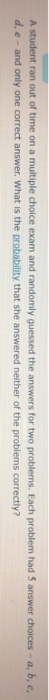A student ran out of time on a multiple choice exam and randomly guessed the answers for two problems. Each problem had 5 answer choices - a, b, c, d. e - and only one correct answer. What is the probability that she answered neither of the problems correctly?

• ### Question Not yet answered Marked out of 1 P Flag question It is known from past...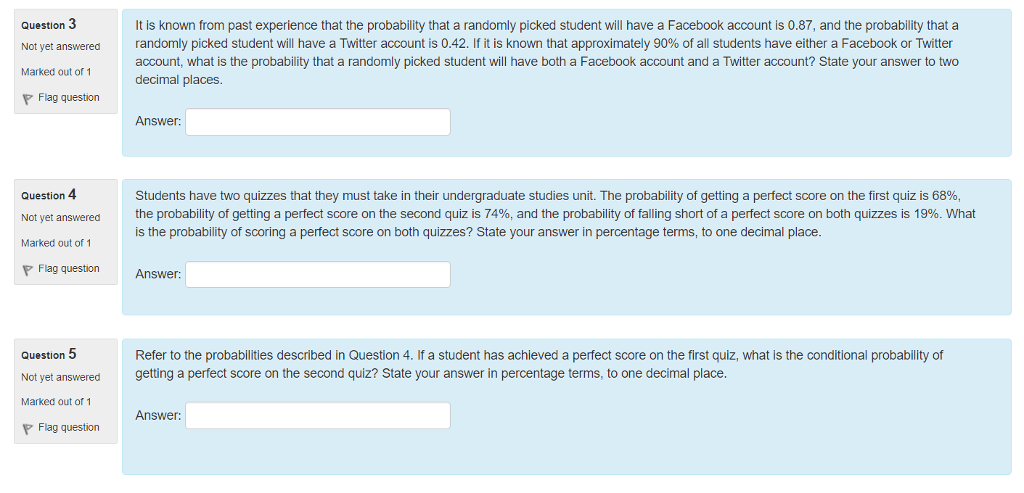Question Not yet answered Marked out of 1 P Flag question It is known from past experience that the probability that a randomly picked student will have a Facebook account is 0.87, and the probability that a randomly picked student will have a Twitter account is 0.42. If it is known that approximately 90% of all students have either a Facebook or Twitter account, what is the probability that a randomly picked student will have both a Facebook account and...

• ### 3. An economics professor went out to dinner one night and observed one of her students...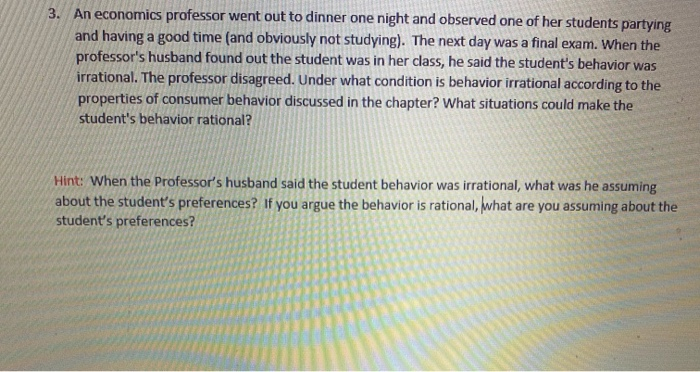3. An economics professor went out to dinner one night and observed one of her students partying and having a good time (and obviously not studying). The next day was a final exam. When the professor's husband found out the student was in her class, he said the student's behavior was irrational. The professor disagreed. Under what condition is behavior irrational according to the properties of consumer behavior discussed in the chapter? What situations could make the student's behavior rational?...

• ### (1 point) An instructor gives his class a set of 18 problems with the information that the next quiz will consist of a random selection or 7 of them. If a student has figured out how to do 12 of the...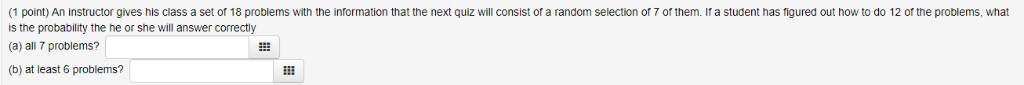(1 point) An instructor gives his class a set of 18 problems with the information that the next quiz will consist of a random selection or 7 of them. If a student has figured out how to do 12 of the problems, what is the probability the he or she will answer correctly (a) all 7 problems? (b) at least 6 problems? (1 point) An instructor gives his class a set of 18 problems with the information that the next...